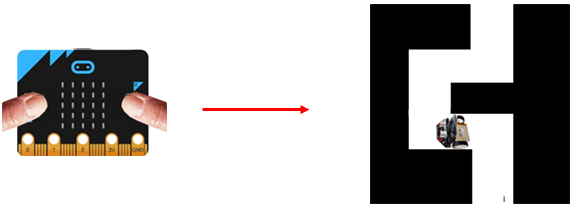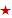Jython
 Deutsch   English

## 6. Learning Robots

 The behavior of many robots is not fixed, but the robots behave according to information they retrieve from a memory, in analogy to our brain. So in some sense, the robot is able to learn. The memory can be filled either by "training"  from a "teacher" or by "self-learning" (learning from personal experience). In the following examples, simple examples are used to show how a robot is taught by a human being. Then it will gain experience and perform a task with the help of its sensors.

Example 1: The robot learns in "teach mode"
Industrial robots are "taught" in a "teach mode" by a specialist (teacher), for example which arm movements are to be carried out to perform a certain task. The teacher usually uses an input system similar to a remote control. The robot is moved to the desired positions one after the other and the respective state is stored.

 In teach mode,  the robot  is remote controlled with a second micro:bit to find its way in a simple labyrinth. Since in this simple situation the straight paths have the same length and are perpendicular to each other, the teacher only has to instruct the robot whether to turn left or right. The robot executes the commands and saves the left or right commands in the list memory. The list is empty at the beginning. When the message LEFT is received, a 0 is added using memory.append(0) , when the message RIGHT is received, a 1 is added with memory.append(1). When the learning process is complete, the robot is put back to the start position by  hand and starts its travel autonomously by clicking button A.Program Teacher (micro:bit): ```#Mr6a.py from microbit import * import radio radio.on() state = "STOP" oldState = "" while True: if button_a.was_pressed(): state = "LEFT" display.show('L') elif button_b.was_pressed(): state = "RIGHT" display.show('R') else: state = "STOP" display.show('S') if oldState != state: radio.send(state) oldState = state sleep(10) ``` #Mq6a.py from microbit import * import radio radio.on() state = "STOP" oldState = "" while True: if button_a.was_pressed(): state = "LEFT" display.show('L') elif button_b.was_pressed(): state = "RIGHT" display.show('R') else: state = "STOP" display.show('S') if oldState != state: radio.send(state) oldState = state sleep(10) ► Copy to clipboard

 Program Robot: ```# Mr6b.html (receiver) from microbit import * from mbrobot import * import radio def fd(): forward() delay(moveTime) stop() def run(): for k in memory: if k == 0: left() delay(turnTime) elif k == 1: right() delay(turnTime) fd() moveTime = 1000 turnTime = 500 radio.on() setSpeed(20) memory= [] while not button_a.was_pressed(): rec = radio.receive() if rec == "LEFT": memory.append(0) left() delay(turnTime) fd() elif rec == "RIGHT": memory.append(1) right() delay(turnTime) fd() else: stop() run() stop() ``` # Mq6b.html (receiver) from microbit import * from mbrobot import * import radio def fd(): forward() delay(moveTime) stop() def run(): for k in memory: if k == 0: left() delay(turnTime) elif k == 1: right() delay(turnTime) fd() moveTime = 1000 turnTime = 500 radio.on() setSpeed(20) memory= [] while not button_a.was_pressed(): rec = radio.receive() if rec == "LEFT": memory.append(0) left() delay(turnTime) fd() elif rec == "RIGHT": memory.append(1) right() delay(turnTime) fd() else: stop() run() exit() ► Copy to clipboard

Explanations of the program code:memory = [] : The list memory is empty at the beginningmemory.append(0): When turning left a 0 is added to the listmemory.append(1): When turning right a 1 is added to the listdef run(): Defines how the labyrinth is to be traversed using the memory

Example 2: The robot learns to traverse a simple labyrinth by using its ultrasonic sensor
By using data from its ultrasonic sensor, the robot learns autonomously to travel the labyrinth consisting of  sections of equal length. It uses the following strategy: It moves forward until it detects a wall nearby. It then turns 90° to the left and examines the surroundings with his ultrasound sensor. If it "sees" a wall nearby, it has chosen the wrong direction and turns 180° to the left and drives to the next wall. It stores in its memory 0 for left and 1 for right turn.
By pressing button A, the robot travels the labyrinth independently using the stored information in its memory.

(Under construktion)

Example 3: The robot learns to traverse a somewhat more complicated labyrinth by using its ultrasonic sensor and a timer

As in example 2, the robot learns where to turn left and right. Since the straight sections have no longer the same length, it starts the timer at the beginning of each section and measures the time to the next wall. In its memory list, it now stores 2 values: The travel time for the corresponding section of road and 0 or 1 for left or right turn.

By pressing button A, the robot travels the labyrinth independently using the stored information in its memory.

(Under construction)